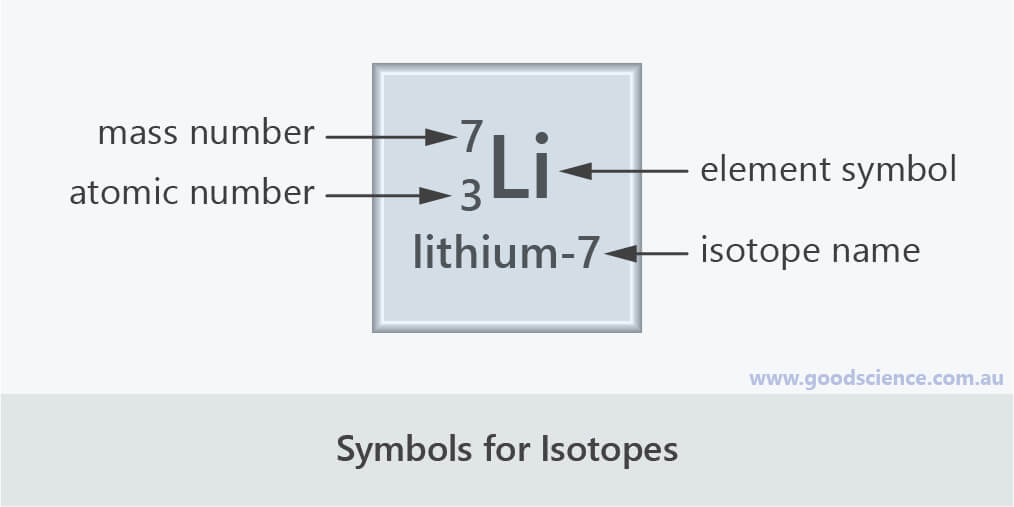Learning Objective

In this lesson we will learn how atoms are defined by the number of protons they contain, as well as the number of neutrons.

Learning Outcomes

By the end of this lesson you will be able to:

• Define atomic number.

• Define mass number.

• Compare isotopes of an element.

BUY THE YEAR 9 CHEMISTRY WORKBOOK

## Atomic Number

• The number of protons in an atom is called the atomic number.• Since the number of protons in the atoms of a particular element is always the same, the atomic number of an element is fixed.
• Examples:
Carbon atoms contain 6 protons, therefore they have the atomic number 6.
Calcium atoms contain 20 protons, therefore they have the atomic number 20.
Lead atoms contain 82 protons, therefore they have the atomic number 82.
• The periodic table arranges elements based on their atomic number.
The atomic number of an element is written above its chemical symbol.
• Since atoms have the same number of electrons as protons, the atomic number also equals the number of electrons in an atom.
However the technical definition is based on the number of protons as it is possible for atoms to gain or lose electrons (which you will learn about a bit later).Carbon atoms contain 6 protons; therefore, carbon has the atomic number 6.

## Mass Number

• The number of protons plus the number of neutrons in an atom is called the mass number.
In other words, the mass number refers to the number of particles in the nucleus of an atom.• Since the number of neutrons in the atoms of a particular element can vary, the mass number of an element can vary.
• Example:
All magnesium atoms have 12 protons, but the number of neutrons can be either 12, 13 or 14.
Magnesium atoms with 12 neutrons have a mass number of 24 (12 + 12).
Magnesium atoms with 13 neutrons have a mass number of 25 (12 + 13).
Magnesium atoms with 14 neutrons have a mass number of 26 (12 + 14).
• The number of neutrons in an atom can be determined by subtracting the number of protons (atomic number) from the mass number.
• Protons and neutrons contribute equally to the mass number of an atom as they both have a mass of one atomic mass unit.
The mass number does not include the number of electrons since their mass is negligible compared to the mass of protons and neutrons.Almost all the mass of an atom is in the nucleus; the mass number of an atom equals the number of nuclear particles (protons and neutrons).

## Isotopes

• Atoms that have the same number of protons but a different number of neutrons are called isotopes.
In other words, isotopes have the same atomic number, but different mass numbers.
Since they have the same number of protons, isotopes are different forms of the same element.
• There are two main ways of depicting isotopes of an element to distinguish between them.
1. Including the isotope’s mass number with the element’s name.
2. Including the isotope’s mass number and atomic number with the element’s symbol.
• Example:
Boron atoms contain 5 protons.
There are two isotopes of boron – one containing 5 neutrons and one containing 6 neutrons.
These isotopes therefore have the atomic number 5 and mass numbers 10 and 11 respectively.
They can be written as boron-10 and boron-11, or asand.
• Almost all elements exist as two or more isotopes.
The proportions of different isotopes are fixed for a particular element, but differ between elements.
For example, naturally occurring boron consists of 20% boron-10 and 80% boron-11; naturally occurring bromine consists of 51% bromine-79 and 49% bromine-81.Boron has two isotopes: boron-10 and boron-11.

## Summary

• The number of protons in an atom is called the atomic number.
Atomic Number  =  Number of Protons
• The number of protons plus the number of neutrons in an atom is called the mass number.
Mass Number  =  Number of Protons  +  Number of Neutrons
• The atomic number of an element is fixed but the mass number can vary.
Atoms that have the same atomic number (number of protons) but different mass numbers (number of neutrons) are called isotopes.The isotope lithium-7 has the atomic number 3 and the mass number 7.

(Header image: Johannes Schneider, Wikimedia Commons)

BUY THE YEAR 9 CHEMISTRY WORKBOOK